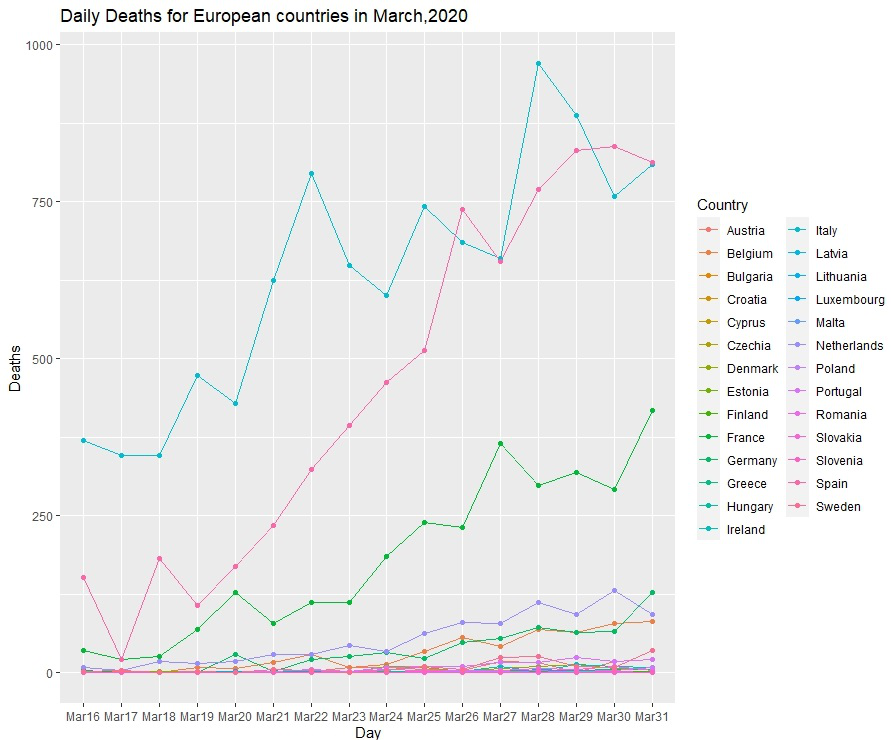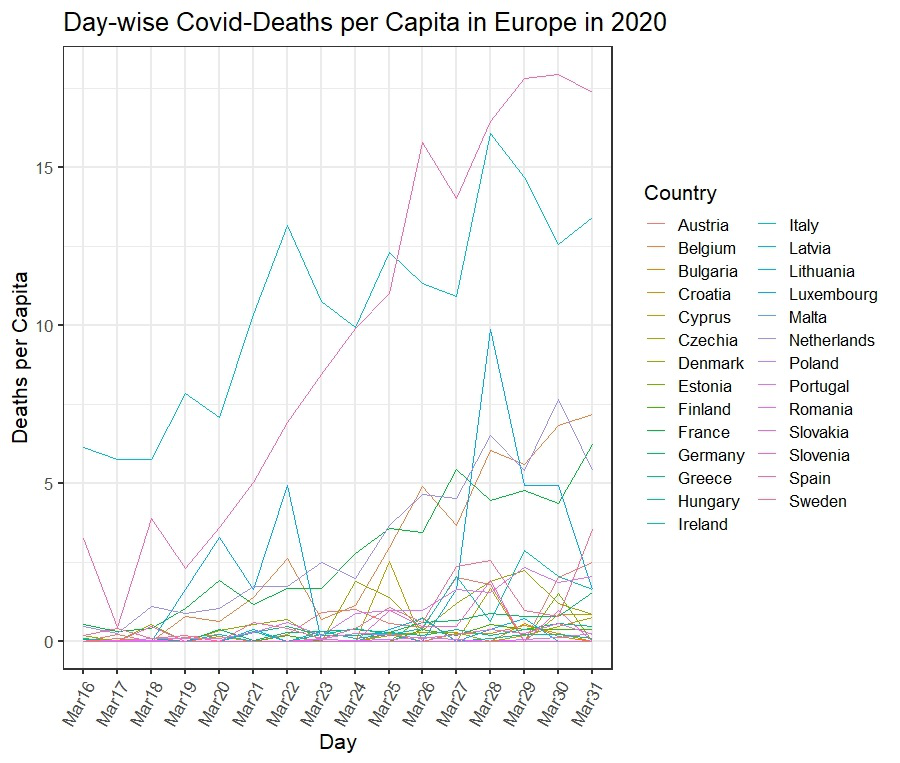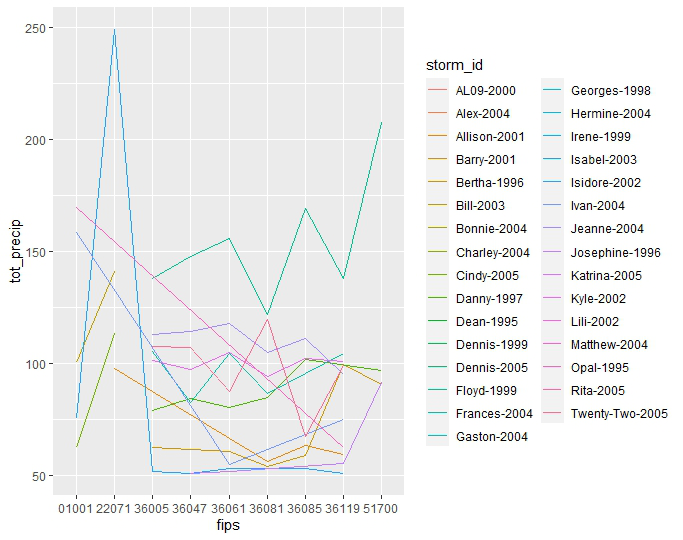# Plotting multiple time series on the same plot using ggplot in R

Time series data is hierarchical data.  It is a series of data associated with a timestamp. An example of a time series is gold prices over a period or temperature range or precipitation during yearly storms. To visualize this data, R provides a handy library called ggplot. Using ggplot, we can see all sorts of plots.  Along with ggplot, R also provides libraries to clean up data and transform or manipulate it to fit our visualization requirements.

This article will look at one dataset from the R datasets and one dataset obtained from a CSV file.

### Dataset 1: EU Covid deaths for March 2020

The dataset gives us the daily death counts from Covid-19 for all European Countries for March 2020. We will plot the number of deaths(y-axis) vs. day(x-axis) for every country.

Plot 1: Daily Death Count

The steps for plotting are as follows:

• Open R Studio and open an R notebook (has more options).
• Save this file as .rmd, preferably in the same folder as your data.
• Select the Working directory to where your data is
• Import all the R libraries
• Read the data from the CSV.
• The data above is spread across columns. To make plotting easier, we need to format the data in the required format.
• Plot data
• Display data

Example:

## R

 `library``(ggplot2)` `library``(reshape2)` `library``(dplyr)`   `covid1 =(``read.csv``(file=``"EUCOVIDdeaths.csv"``,header=``TRUE``)[,-``c``(2)])`   `head``(covid1)`     `covid_deaths <- ``melt``(covid1,id.vars=``c``(``"Country"``),value.name=``"value"``,` `                     ``variable.name=``"Day"``)`   `head``(covid_deaths)`   `covid_plot <- ``ggplot``(data=covid_deaths, ``aes``(x=Day, y=value, group = Country, ` `                                            ``colour = Country))` `+ ``geom_line``() +``labs``(y= ``"Deaths"``, x = ``"Day"``)` `covid_plot + ``ggtitle``(``"Daily Deaths for European countries in March,2020"``)+``geom_point``()`   `covid_plot`

Output:Daily Deaths timeseries plot with points

Plot 2: Plotting covid deaths per capita.

We will be using the same data as the previous example. But here we will be dealing with per capita data.

## R

 `library``(ggplot2)` `library``(reshape2)` `library``(dplyr)`   `covid1 =(``read.csv``(file=``"EUCOVIDdeaths.csv"``,header=``TRUE``)[,-``c``(2)])`   `head``(covid1)`   `covid_perCapita <- covid1[,``c``(2:17)] / covid\$PopulationM`   `covid_perCapita\$Country <- covid1\$Country`   `head``(covid_perCapita)`   `covid_perCapita_deaths <- ``melt``(covid_perCapita,id.vars=``c``(``"Country"``),` `                               ``value.name=``"value"``, variable.name=``"Day"``)`   `covidPerCapitaPlot <- ``ggplot``(data=covid_perCapita_deaths,` `   ``aes``(x=Day, y=value, group = Country, colour = Country)) + ``geom_line``() ` `   ``+``labs``(y= ``"Deaths per Capita"``, x = ``"Day"``)  + ``theme_bw``(base_size = 16) ` `+ ``theme``(axis.text.x=``element_text``(angle=60,hjust=1))` `+ ``ggtitle``(``"Day-wise Covid-Deaths per Capita in Europe in 2020"``)`   `covid_perCapitaPlot`

Output:Capita Plot

### Dataset 2: Rainfall for US counties during tropical storms.

First install the package: hurricaneexposuredata

Before installing the package, please check the R version. To check the R version in RStudio go to Tools -> Global Options. In the window that opens, in the Basic Tab, we see the R version.

#If the R version is the greater than 4

install.packages(“hurricaneexposuredata”)

#For R versions lower than 4.0, please install this way

install.packages(‘hurricaneexposuredata’, repos=’https://geanders.github.io/drat/’, type=’source’)

Example:

## R

 `library``(hurricaneexposuredata)` `library``(hurricaneexposure)`   `rain_data <- ``county_rain``(counties = ``c``(``"01001"``,``"36005"``, ``"36047"``,` `                                      ``"36061"``,``"36085"``, ``"36081"``,` `                                      ``"36119"``,``"22071"``, ``"51700"``), ` `              ``start_year = 1995, end_year = 2005, rain_limit = 50, ` `                         ``dist_limit = 500, days_included = ``c``(-1, 0, 1))`   `ggplot``(data = rain_data, ``aes``(x=fips, y=tot_precip, group=storm_id,` `                             ``color=storm_id)) + ``geom_line``()`

Output:Whether you're preparing for your first job interview or aiming to upskill in this ever-evolving tech landscape, GeeksforGeeks Courses are your key to success. We provide top-quality content at affordable prices, all geared towards accelerating your growth in a time-bound manner. Join the millions we've already empowered, and we're here to do the same for you. Don't miss out - check it out now!

Previous
Next